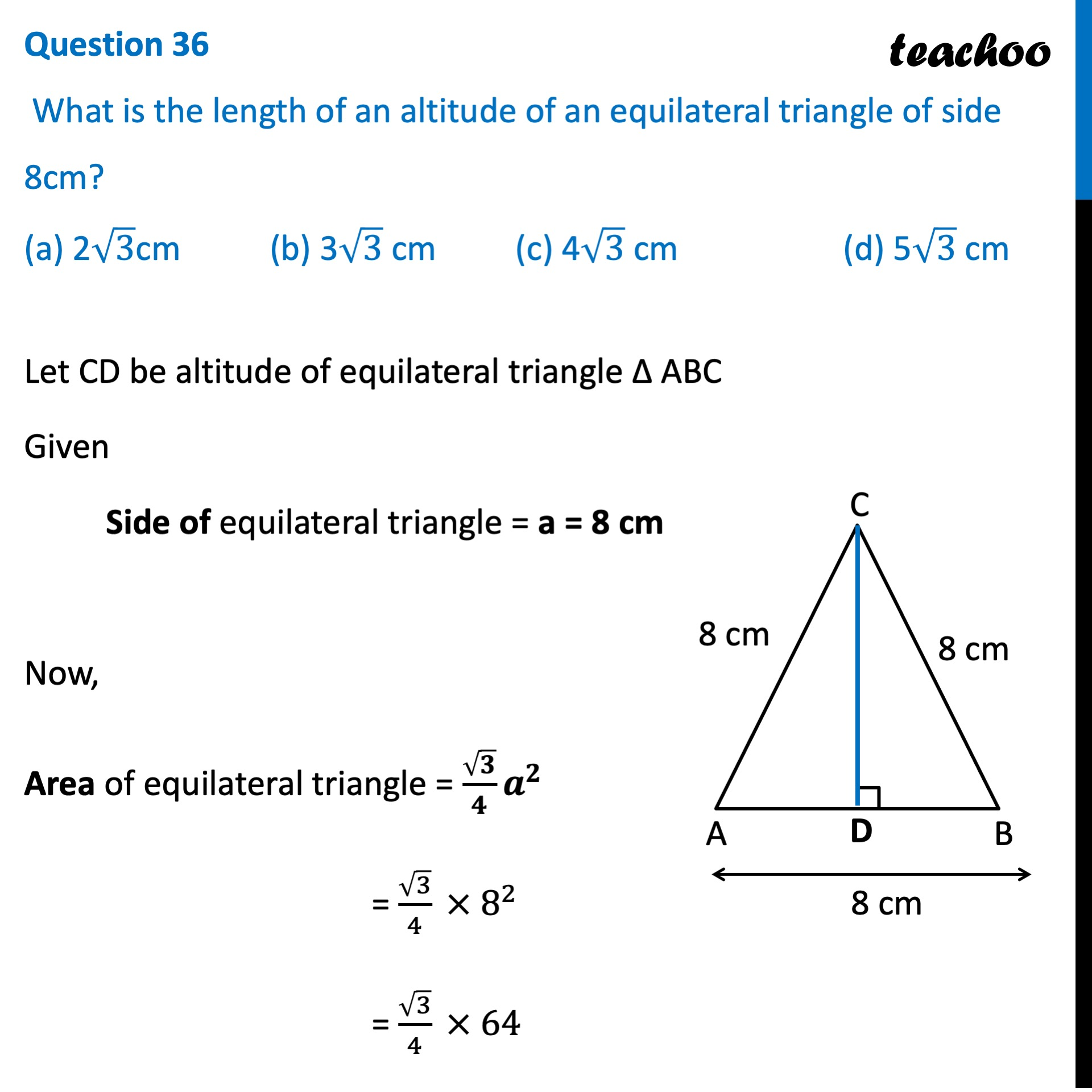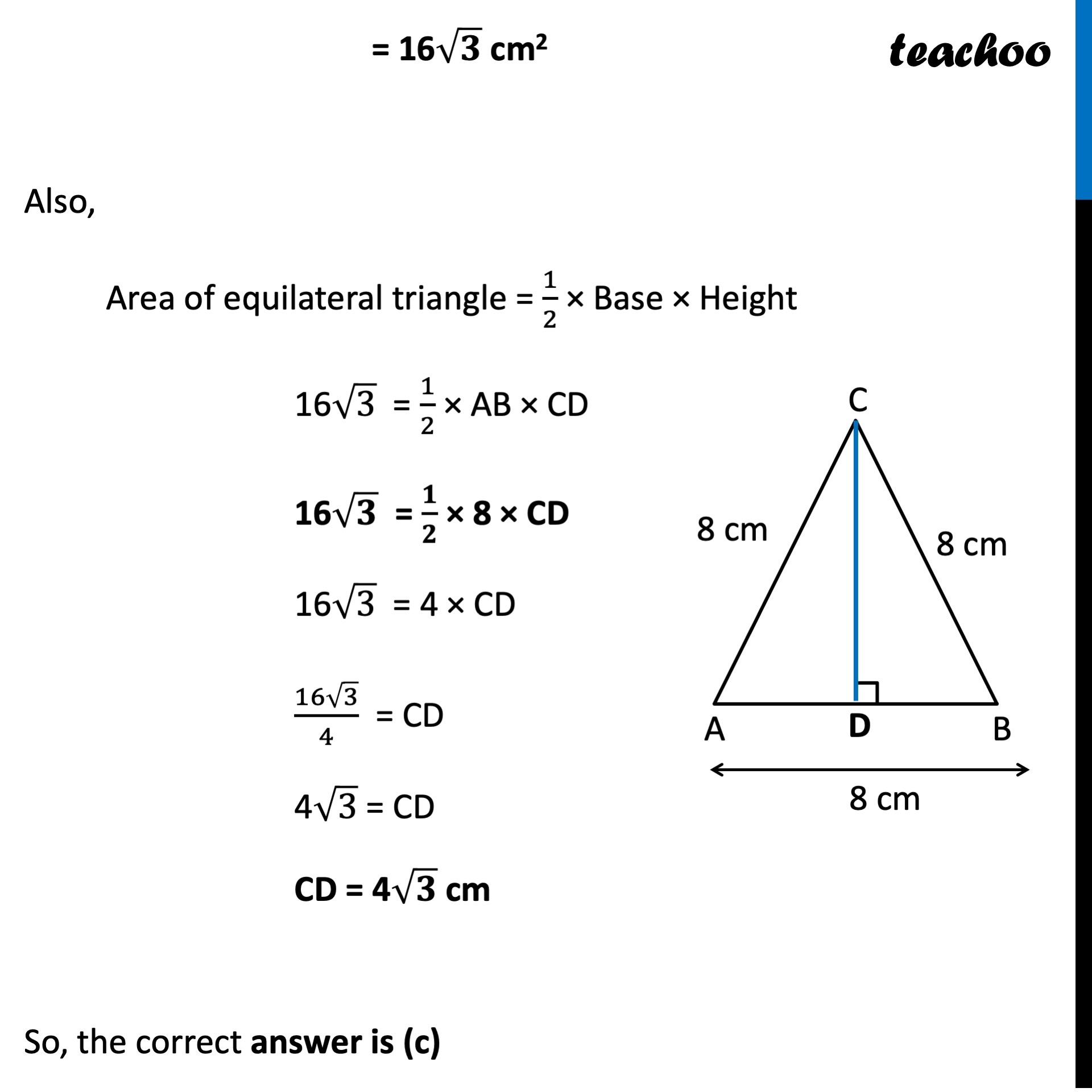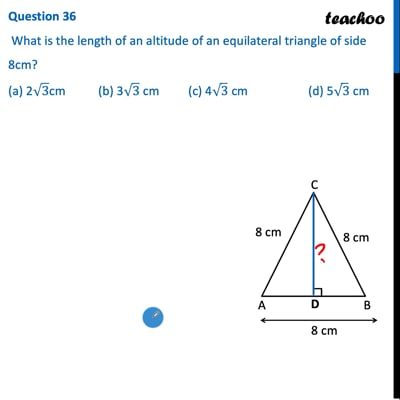CBSE Class 10 Sample Paper for 2022 Boards - Maths Basic [MCQ]

Class 10
Solutions of Sample Papers for Class 10 Boards

## (a) 2√3cm   (b) 3√3 cm   (c) 4√3 cm   (d) 5√3 cmThis video is only available for Teachoo black users

Introducing your new favourite teacher - Teachoo Black, at only ₹83 per month

### Transcript

Question 36 What is the length of an altitude of an equilateral triangle of side 8cm? (a) 2√3cm (b) 3√3 cm (c) 4√3 cm (d) 5√3 cm Let CD be altitude of equilateral triangle Δ ABC Given Side of equilateral triangle = a = 8 cm Now, Area of equilateral triangle = √𝟑/𝟒 𝒂^𝟐 = √3/4 × 8^2 = √3/4 × 64 = 16√𝟑 cm2 Also, Area of equilateral triangle = 1/2 × Base × Height 16√3 = 1/2 × AB × CD 16√𝟑 = 𝟏/𝟐 × 8 × CD 16√3 = 4 × CD (16√3)/4 = CD 4√3 = CD CD = 4√𝟑 cm So, the correct answer is (c)